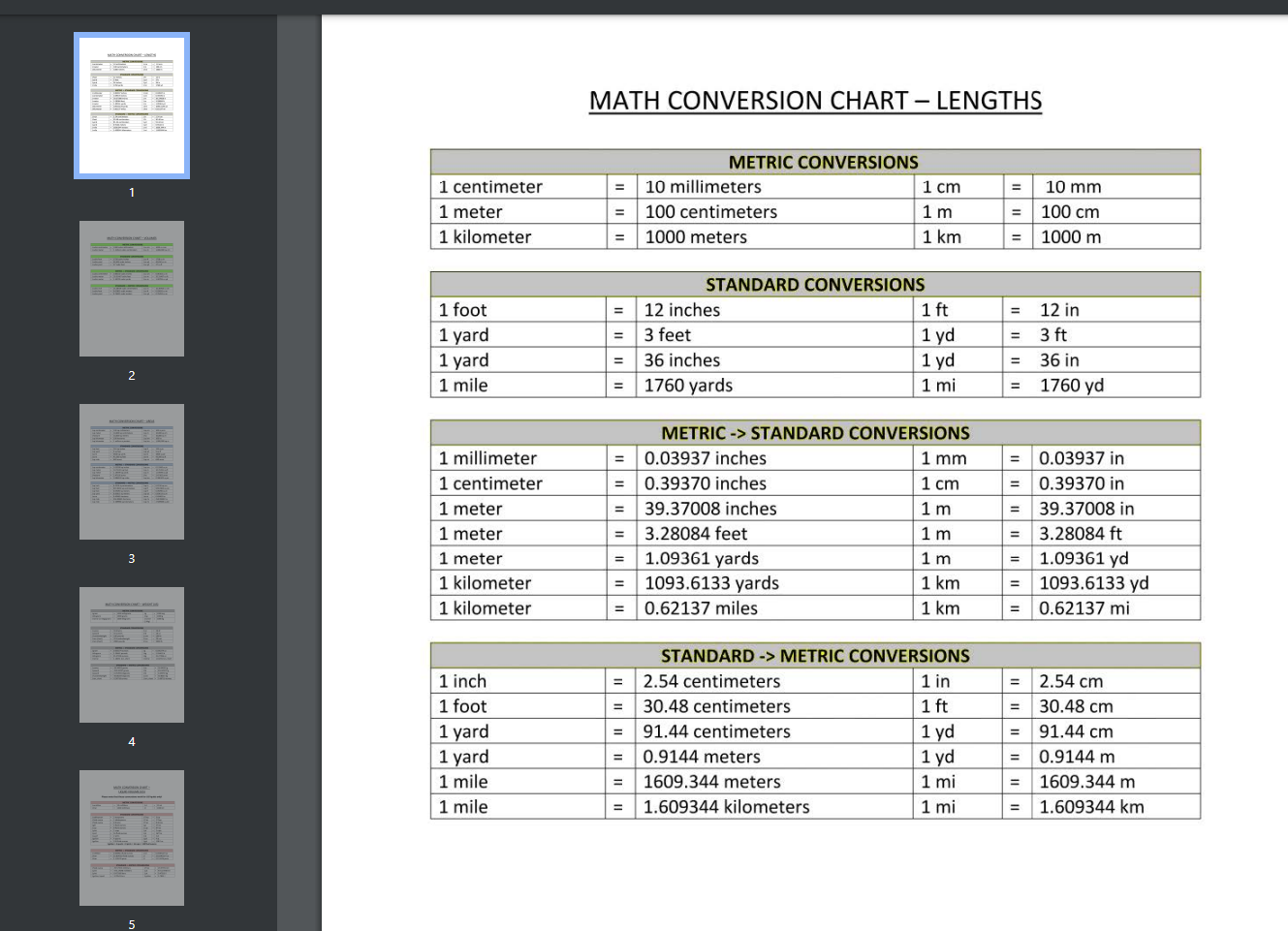Math Metric System Conversion Reference ChartKlicken Sie auf das Bild zum Vergrößern / Klicken Sie auf die Schaltfläche unten, um mehr Bilder zu sehen

Speichern, ausfüllen, drucken, fertig!How to make a Math Metric System Conversion Reference Chart? Download this Math Metric System Conversion Reference Chart template that will perfectly suit your needs!

Verfügbare Gratis-Dateiformate:

.pdf

• Dieses Dokument wurde von einem Professional zertifiziert
• 100% anpassbar

Benutzer-Bewertung: 8

Malware- und virenfrei. Gescannt von:Education Bildung metric system conversion metrische Systemkonvertierung math Mathematik mathematics Mathematik mathematical mathematisch Metric Metrisch Conversion Umwandlung sine Sinus cosine Kosinus metric conversion metrische Umrechnung math conversion mathematische Konvertierung math conversion chart Matheumwandlungstabelle math conversions chart Matheumwandlungstabelle conversion chart math Umrechnungstabelle math tangent sin cos tan calculator sin cos tan table formulas sin cos tan csc sec cot sin cos tan calculator triangle sin formula sine cosine tangent trigonome functions inverse trigonomet functions law of sines cosecant coversine versine secant exsecant haversine formula

How to make a Math Metric System Conversion Reference Chart? Download this Math Metric System Conversion Reference Chart template that will perfectly suit your needs!

Mathematicians regularly need spreadsheets, worksheets or forms, and often more than any other industry. Therefore, we support you by providing this Math Metric System Conversion Reference Chart template, which will save your time, cost and efforts and help you to reach the next level of success in your studies, work or business!

This blank Math Metric System Conversion Reference Chart is intuitive, ready-to-use and structured in a smart way. Try it now and let this mathematics template inspire you. We certainly encourage to use this Math Metric System Conversion Reference Chart for your own good and are confident it will fit your needs

Our site is often updated with new templates.